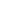#MTH-1005 Working with Fractions is a Course

## MTH-1005 Working with Fractions

Self-paced

### Full course description

Course Description

In this course, you will learn about fractions. You’ll discover how to convert improper fractions to mixed fractions. You’ll also find out how to simplify fractions and convert between fractions, decimals, and percentages. Finally, you’ll learn to add, subtract, multiply, and divide fractions and solve word problems.

By the end of this course, you will be able to

• Convert between improper fractions and mixed numbers

• Simplify fractions

• Convert between fractions, decimals, and percentages

• Add, subtract, multiply, and divide fractions

• Understand reciprocal fractions

• Add, subtract, and multiply mixed fractions

• List the steps to solve word problems

Estimated completion time (hours): 1.4